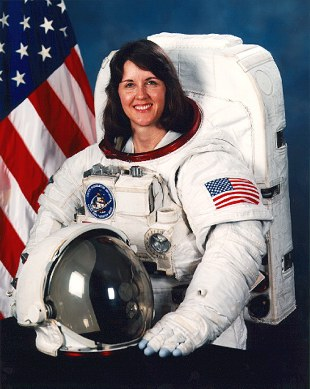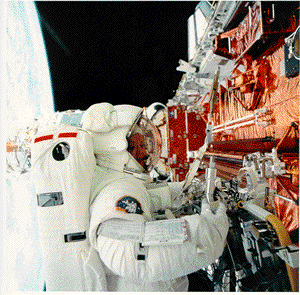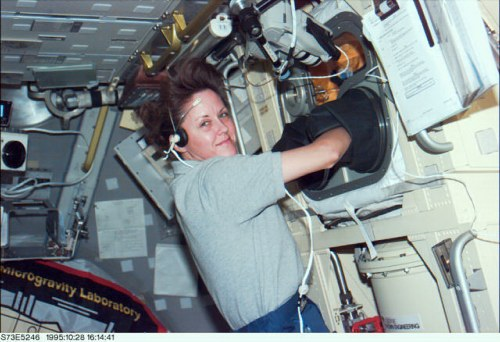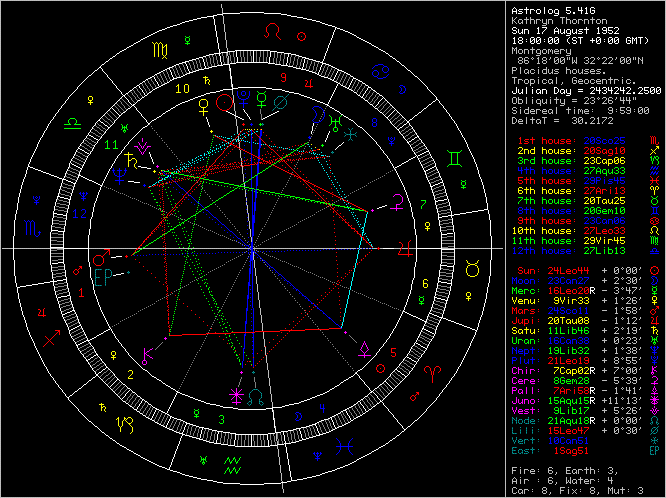Kathryn ThorntonEL61/Sun conjunction

>>Kathryn Ryan Cordell Thornton (Ph.D.) (born August 17, 1952 in Montgomery, Alabama) is an American scientist and a former NASA astronaut with over 975 hours in space, including 21 hours of extravehicular activity. She is currently the Associate Dean for Graduate Programs in the University of Virginia School of Engineering and Applied Science.
Education and organizations
Graduated from Sidney Lanier High School, Montgomery, Alabama, in 1970; received a bachelor of science degree in physics from Auburn University in 1974, a master of science degree in physics from the University of Virginia in 1977, and a doctorate of philosophy in physics from the University of Virginia in 1979.
Member of the American Physical Society, American Association for the Advancement of Science, Sigma Xi, Phi Kappa Phi, and Sigma Pi Sigma.

Experience
After Dr. Thornton earned her Ph.D. at the University of Virginia in 1979, she was awarded a NATO Postdoctoral Fellowship to continue her research at the Max Planck Institute for Nuclear Physics in Heidelberg, Germany. In 1980, she returned to Charlottesville, Virginia, where she was employed as a physicist at the U.S. Army Foreign Science and Technology Center.

NASA experience
Selected by NASA in May 1984, Dr. Thornton became an astronaut in July 1985. Her technical assignments have included flight software verification in the Shuttle Avionics Integration Laboratory (SAIL), serving as a team member of the Vehicle Integration Test Team (VITT) at Kennedy Space Center, and as a spacecraft communicator (CAPCOM). A veteran of three space flights, Dr. Thornton flew on STS-33 in 1989, STS-49 in 1992, and STS-61 in 1993. She has logged over 975 hours in space, including more than 21 hours of extravehicular activity (EVA).Dr. Thornton was a mission specialist on the crew of STS-33 which launched at night from Kennedy Space Center, Florida, on November 22, 1989, aboard the Space Shuttle Discovery. The mission carried Department of Defense payloads and other secondary payloads. After 79 orbits of the Earth, this five-day mission concluded on November 27, 1989, at Edwards Air Force Base, California.
On her second flight, Dr. Thornton served on the crew of STS-49, May 7-16, 1992, on board the maiden flight of the new Space Shuttle Endeavour. During the mission the crew conducted the initial test flight of Endeavour, performed a record four EVA's (space walks) to retrieve, repair and deploy the International Telecommunications Satellite (INTELSAT), and to demonstrate and evaluate numerous EVA tasks to be used for the assembly of Space Station Freedom.
Dr. Thornton was one of two EVA crew members who evaluated Space Station assembly techniques on the fourth EVA. STS-49 logged 213 hours in space and 141 Earth orbits prior to landing at Edwards Air Force Base, California.
On her third flight, Dr. Thornton was a mission specialist EVA crew member aboard the Space Shuttle Endeavour on the STS-61 Hubble Space Telescope (HST) servicing and repair mission. STS-61 launched at night from the Kennedy Space Center, Florida, on December 2, 1993. During the 11-day flight, the HST was captured and restored to full capacity through a record five space walks by four astronauts (including her.) After having travelled 4,433,772 miles in 163 orbits of the Earth, the crew of Endeavour returned to a night landing at the Kennedy Space Center on December 13, 1993. Then, after Expedition 14, Sunita Williams surpassed her for woman with the most spacewalks.
From October 20 to November 5, 1995, Dr. Thornton served aboard Space Shuttle Columbia on STS-73, as the payload commander of the second United States Microgravity Laboratory mission. The mission focused on materials science, biotechnology, combustion science, the physics of fluids, and numerous scientific experiments housed in the pressurized Spacelab module. In completing her fourth space flight, Dr. Thornton orbited the Earth 256 times, traveled over 6 million miles, and logged a total of 15 days, 21 hours, 52 minutes and 21 seconds in space.Dr. Thornton left NASA on August 1, 1996, to join the faculty of the University of Virginia School of Engineering. She also serves as the director of UVA's Center for Science, Mathematics, and Engineering Education.

Personal
Thornton is married to Stephen T. Thornton, Ph.D., of Oak Ridge, Tennessee. She has two stepsons and three daughters. She enjoys scuba diving and skiing.<<

http://en.wikipedia.org/wiki/Kathryn_Thornton

_______________________________

Assuming noon in Montgomery, Alabama (Lat32n22 - Lon86w18), 18:00 UT

Using RIYAL 3.1

Astrological Setting (Tropical - Placidus)RIYAL  Sun August 17 1952  UT 18h00m00s  Lat32n22  Lon86w18   SORT ALL

Planet    Longit.    Latit.     Declin.   Const.

VS2      =  0Pi12 r    7n15    4s37   Aqr
PJ30     =  0Li21      5s03    4s46   Vir
XA255    =  0Ta31 r   12s28    0s02   Cet
Pholus   =  0Aq42 r    1s16   21s14   Cap
Chaos    =  0Ar45 r    9s21    8s16   Cet
RP120    =  1Sc10     11s14   22s22   Vir
QB243    =  1Le24      3n12   22n58   Cnc
Amycus   =  1Ta55 r   13n04   24n22   Psc
OO67     =  2Sa06 r   19n44    1s13   Ser
MS4      =  2Sc14      0s38   12s51   Vir
UJ438    =  2Sa44      1s06   21s48   Sco
SB60     =  2Cp46 r    2s28   25s53   Sgr
QB1      =  2Aq53 r    1s50   21s18   Cap
CF119    =  2Ge57     17n20   37n42   Per
Thereus  =  3Vi18     14s49    3s32   Sex
DA62     =  3Ta36 r    3s53    9n04   Cet
Cyllarus =  4Sc13      3n22    9s46   Vir
CY118    =  4Ca13     23s50    0s27   Ori
GB32     =  4Le27     11s09    8n19   Cnc
GV9      =  5Le25     17s52    1n33   Hya
CO1      =  5Ar30 r   19s30   15s41   Cet
TO66     =  5Aq35 r   21s44   39s49   Mic
GM137    =  5Le48      7n38   26n13   Cnc
CZ118    =  5Le57     18n01   36n10   Lyn
SA278    =  6Pi25 r    3n57    5s30   Aqr
SQ73     =  6Le33     15n49   33n53   Lyn
RZ214    =  6Cp41 r   18n52    4s26   Sct
Varuna   =  6Ta44 r   15s17    0s42   Cet
Chiron   =  7Cp02 r    7n00   16s16   Sgr
CR105    =  7Ge05     20s13    1n33   Tau
QF6      =  7Li15     17n12   12n55   Vir
HB57     =  7Le20     14s24    4n30   Hya
WL7      =  7Sc32      6s30   20s10   Vir
Eris     =  8Ar07 r   24s19   19s03   Cet
Quaoar   =  8Li29      0n09    3s13   Vir
RN43     =  8Sa55 r   17n33    4s26   Oph
Apogee   =  9Le01      1n09   19n07   Cnc
Venus    =  9Vi33      1n26    9n19   Leo
GQ21     =  9Le34     11s50    6n26   Hya
Bienor   =  9Ge55     20n23   42n04   Per
Asbolus  = 10Ca13     17n08   40n08   Aur
VU2      = 10Sc31      5s39   20s21   Lib
Vertex   = 10Ca51
UR163    = 11Aq44 r    0n09   17s08   Cap
Saturn   = 11Li46      2n19    2s31   Vir
Typhon   = 11Ar53 r    0n50    5n28   Psc
XR190    = 11Ta56 r   28n36   42n16   And
TD10     = 12Sc13      3n43   11s57   Lib
TY364    = 12Aq22 r    3n24   13s50   Aqr
Hylonome = 12Ta41 r    2s52   12n55   Ari
RR43     = 12Aq51 r   22s32   38s23   Gru
Crantor  = 13Ar08 r   12s41    6s30   Cet
RD215    = 13Cp16 r   25n30    2n36   Ser
KF77     = 13Ca46      4n15   26n58   Gem
96PW     = 13Vi59     11n02   16n27   Leo
FP185    = 14Ca03     29s37    6s46   Mon
RM43     = 14Aq10 r   23s21   38s43   Gru
FZ53     = 14Pi20 r   23n56   15n54   Peg
MW12     = 14Li41      4n40    1s29   Vir
Elatus   = 14Pi56 r    6s10   11s37   Aqr
DH5      = 15Aq08 r    8n41    7s59   Aqr
KX14     = 15Vi29      0s20    5n25   Leo
VQ94     = 15Ar32 r   43s59   34s05   For
WN188    = 15Cp49 r    7n03   15s30   Sgr
RZ215    = 16Sc19     23s34   39s05   Cen
Ixion    = 16Li19     15n43    8n06   Vir
VR130    = 16Sc20      2n34   14s16   Lib
Mercury  = 16Le20 r    3s47   12n20   Cnc
FZ173    = 16Ca26     12n10   34n31   Gem
Echeclus = 16Ca34      3s48   18n39   Gem
Uranus   = 16Ca38      0n23   22n47   Gem
OM67     = 16Sa49 r   22s03   44s42   Sco
LE31     = 17Sa03 r   11n54   10s58   Oph
CO104    = 17Pi05 r    0s21    5s26   Aqr
Nessus   = 17Ta14 r    4n07   20n56   Ari
Ceto     = 19Ar31 r   10s44    2s18   Cet
Neptune  = 19Li32      1n38    6s08   Vir
YQ179    = 19Ta38     18s24    0s06   Tau
Jupiter = 20Ta08      1s12   16n38   Ari
(Ascend)   = 20Sc25      0n00   17s51   Lib
CC22     = 21Aq02 r    0s53   15s20   Cap
TC302    = 21Pi14 r   20s55   22s35   Cet
Pluto    = 21Le19      8n55   22n50   Leo
Okyrhoe  = 21Ar28 r    6s41    2n10   Cet
Node     = 21Aq33      0n00   14s19   Cap
TL66     = 21Aq42 r   23n45    8n16   Equ
BL41     = 22Sc24      9s43   27s44   Lib
Teharonhi= 22Sa55 r    1s40   24s56   Oph
CE10     = 23Li13     30s02   36s31   Cen
EL61     = 23Le21     11n09   24n14   Leo
(Moon)     = 23Ca27      2n30   23n52   Gem
OP32    = 23Sc37     22n15    2n55   Ser
Chariklo = 23Sa53 r   13s33   36s50   Sco
BU48     = 24Aq01 r    3s06   16s27   Cap
Mars     = 24Sc11      1s58   20s44   Lib
PN34     = 24Le19      7s13    6n36   Hya
XX143    = 24Vi21      6n13    7n57   Vir
FY9     = 24Ca39     17n24   38n16   Lyn
Sun      = 24Le44      0n00   13n17   Leo
Sedna    = 24Ar50 r   10s29    0s09   Cet
Pelion   = 25Ca29      1s58   19n07   Gem
TX300    = 25Cp49 r   12s51   33s35   Sgr
RG33     = 26Ca01     33n28   53n32   UMa
AZ84     = 26Ta09      3n55   23n06   Tau
XZ255    = 26Vi55      2n26    3n27   Vir
Huya     = 27Ca12     12s04    8n52   CMi
(Midheav)  = 27Le34      0n00   12n19   Leo
AW197    = 28Ge10     12n29   35n55   Aur
UX25     = 28Aq32 r   16n34    3n35   Peg
OX3      = 28Sc36      1s26   21s15   Lib
Deucalion= 28Le42      0n21   12n16   Leo
Pylenor  = 29Ca01      1s46   18n38   Cnc
Logos    = 29Ge23      1s59   21n28   Ori
Orcus    = 29Ge25      0s07   23n20   Tau
QD112    = 29Ge33     14n13   37n40   Aur
RL43     = 29Li39      9n17    2s39   Vir
GZ32     = 29Ta41     11s27    8n53   Tau
PB112    = 29Sa45 r   15s32   38s59   CrA
PA44     = 29Pi47 r    2n33    2n16   Psc
_____________________________

Focused Minor Planets

EL61     = 23 Le 21    (The Courage to make a Choice, Overcoming a Fear)
Sun       = 24 Le 44
TL66     = 21 Aq 42 r

Mars     = 24 Sc 11        Square
OP32   = 23 Sc 37

Sedna   = 24 Ar 50 r      Trine
Chariklo = 23 Sa 53 r

Quaoar =   8 Li 29         Semisquare

XX143   = 24 Vi 21        Semisextile
_____________________

OP32    = 23 Sc 37
Mars      = 24 Sc 11
Sun       = 24 Le 44        Square

Makemake = 24 Ca 39  Trine
Pelion    = 25 Ca 29

Sedna    = 24 Ar 50 r     Quincunx

Quaoar  =   8 Li 29        Semisquare

XX143    = 24 Vi 21       Sextile

Chariklo  = 23 Sa 53 r   Semisextile
_____________________

Ixion     = 16 Li 19

Mercury  = 16 Le 20 r    Sextile
OM67    = 16 Sa 49 r

Uranus = 16 Ca 38        Square
FZ173    = 16 Ca 26
Echeclus = 16 Ca 34

OO67     =    2 Sa 06 r     Semisquare

RZ215    =  16 Sc 19       Semisextile
KX14     =  15 Vi 29
_____________________

Eris      =   8 Ar 07 r
Quaoar  =  8 Li 29
Venus  =    9 Vi 33         Quincunx

TL66    =  21 Aq 42 r      Semisquare

GQ21   =    9 Le 34         Trine
RN43   =    8 Sa 55 r

Radamantu =  8 Ca 15   Square

Bienor  =    9 Ge 55        Sextile
_____________________

TL66     = 21 Aq 42 r
Node     = 21 Aq 33
Pluto    = 21 Le 19

Jupiter = 20 Ta 08        Square

Eris       =   8 Ar 07 r      Semisquare
Chiron   =   7 Cp 02 r

TC302  = 21 Pi 14 r       Semisextile
_____________________

Typhon   = 11 Ar 53 r
Saturn   = 11 Li 46

TY364    = 12 Aq 22 r    Sextile
RR43     = 12 Aq 51 r
UR163    = 11 Aq 44 r

XR190    = 11 Ta 56 r    Semisextile
Hylonome = 12 Ta 41 r

TD10      =  12 Sc 13      Quincunx

Asbolus  =  10 Ca 13     Square
_____________________

Ceto      =  19 Ar 31 r
Neptune  = 19 Li 32

YQ179   = 19 Ta 38        Semisextile
_______________________________
_______________________________

RIYAL  Sun August 17 1952  UT 18h00m00s  Lat32n22  Lon86w18   SORT ALL

Planet    Longit.

Mars    =  0Sc07
PN34     =  0Le14
XX143    =  0Vi16
FY9      =  0Ca34
Sun      =  0Le40
Sedna    =  0Ar45 r
Pelion   =  1Ca24
TX300    =  1Cp44 r
RG33     =  1Ca56
AZ84     =  2Ta04
XZ255    =  2Vi50
Huya     =  3Ca08
(Midheav)  =  3Le29
AW197    =  4Ge05
UX25     =  4Aq27 r
OX3      =  4Sc31
Deucalion=  4Le37
Pylenor  =  4Ca56
Logos    =  5Ge18
Orcus    =  5Ge21
QD112    =  5Ge28
RL43     =  5Li34
GZ32     =  5Ta36
PB112    =  5Sa40 r
PA44     =  5Pi42 r
VS2      =  6Aq07 r
PJ30     =  6Vi16
XA255    =  6Ar26 r
Pholus   =  6Cp37 r
Chaos    =  6Pi40 r
RP120    =  7Li05
QB243    =  7Ca19
Amycus   =  7Ar50 r
OO67    =  8Sc02 r
MS4      =  8Li10
UJ438    =  8Sc39
SB60     =  8Sa41 r
QB1      =  8Cp48 r
CF119    =  8Ta52
Thereus  =  9Le13
DA62     =  9Ar32 r
Cyllarus = 10Li08
CY118    = 10Ge08
GB32     = 10Ca22
GV9      = 11Ca20
CO1      = 11Pi25 r
TO66     = 11Cp30 r
GM137    = 11Ca43
CZ118    = 11Ca52
SA278    = 12Aq20 r
SQ73     = 12Ca29
RZ214    = 12Sa36 r
Varuna   = 12Ar39 r
Chiron   = 12Sa57 r
CR105    = 13Ta00
QF6      = 13Vi10
HB57     = 13Ca15
WL7      = 13Li27
Eris     = 14Pi02 r
Quaoar   = 14Vi24
RN43     = 14Sc50 r
Apogee   = 14Ca57
Venus    = 15Le28
GQ21     = 15Ca29
Bienor   = 15Ta50
Asbolus  = 16Ge09
VU2      = 16Li26
Vertex   = 16Ge46
UR163    = 17Cp39 r
Saturn   = 17Vi41
Typhon   = 17Pi48 r
XR190    = 17Ar51 r
TD10     = 18Li08
TY364    = 18Cp17 r
Hylonome = 18Ar36 r
RR43     = 18Cp46 r
Crantor  = 19Pi04 r
RD215    = 19Sa11 r
KF77     = 19Ge41
96PW     = 19Le54
FP185    = 19Ge59
RM43     = 20Cp05 r
FZ53     = 20Aq15 r
MW12     = 20Vi36
Elatus   = 20Aq51 r
DH5      = 21Cp03 r
KX14     = 21Le24
VQ94     = 21Pi27 r
WN188    = 21Sa44 r
RZ215    = 22Li14
Ixion    = 22Vi14
VR130    = 22Li16
Mercury  = 22Ca16 r
FZ173    = 22Ge21
Echeclus = 22Ge29
Uranus   = 22Ge33
OM67     = 22Sc44 r
LE31     = 22Sc58 r
CO104    = 23Aq00 r
Nessus   = 23Ar09 r
Ceto     = 25Pi26 r
Neptune  = 25Vi28
YQ179    = 25Ar33
Jupiter  = 26Ar03
(Ascend)   = 26Li20
CC22     = 26Cp57 r
TC302    = 27Aq09 r
Pluto    = 27Ca14
Okyrhoe  = 27Pi23 r
Node     = 27Cp28
TL66     = 27Cp38 r
BL41     = 28Li19
Teharonhi= 28Sc50 r
CE10     = 29Vi08
EL61    = 29Ca16
(Moon)     = 29Ge22
OP32     = 29Li32
Chariklo = 29Sc48 r
BU48     = 29Cp56 r
___________________

Focused Minor Planets

EL61     =  29 Ca 16     (The Courage to make a Choice, Overcoming a Fear)
Sun       =    0 Le 40
TL66     = 27 Cp 38 r

Mars     =   0 Sc 07        Square
OP32   = 29 Li 32

Sedna   =   0 Ar 45 r      Trine
Chariklo =  29 Sc 48 r

Quaoar   =  14 Vi 24     Semisquare

XX143   =    0 Vi 16       Semisextile
_____________________

OP32    = 29 Li 32
Mars      =   0 Sc 07

Sun       =    0 Le 40       Square

Makemake = 0 Ca 34   Trine
Pelion    =    1 Ca 24

Sedna    =    0 Ar 45 r    Quincunx

Quaoar  = 14 Vi 24       Semisquare

XX143    =   0 Vi 16       Sextile

Chariklo =  29 Sc 48 r   Semisextile
_____________________

Ixion      =  22 Vi 14

Mercury  = 22 Ca 16 r    Sextile
OM67     =  22 Sc 44 r

Uranus  =  22 Ge 33       Square
FZ173    =  22 Ge 21
Echeclus = 22 Ge 29

OO67     =    8 Sc 02 r      Semisquare

RZ215    = 22 Li 14          Semisextile
KX14     =  21 Le 24
_____________________

Eris       =   14 Pi 02 r
Quaoar =  14 Vi 24

Venus   =  15 Le 28         Quincunx

TL66     =   27 Cp 38 r     Semisquare

GQ21    =   15 Ca 29       Trine
RN43    =   14 Sc 50 r

Radamantu = 14 Ge 10  Square

Bienor   =   15 Ta 50        Sextile
_____________________

TL66     = 27 Cp 38 r
Node     = 27 Cp 28
Pluto    =  27 Ca 14

Jupiter =  26 Ar 03       Square

Eris       =  14 Pi 02 r    Semisquare
Chiron   =  12 Sa 57 r

TC302  =   27 Aq 09 r   Semisextile
_____________________

Typhon   =  17 Pi 48 r
Saturn   = 17 Vi 41

TY364    =  18 Cp 17 r    Sextile
RR43     =  18 Cp 46 r
UR163    = 17 Cp 39 r

XR190    = 17 Ar 51 r     Semisextile
Hylonome = 18 Ar 36 r

TD10      =  18 Li 08        Quincunx

Asbolus  =  16 Ge 09      Square
_____________________

Ceto       =   25 Pi 26 r
Neptune = 25 Vi 28

YQ179    =  25 Ar 33       Semisextile
_____________________________________________

Posted to Centaurs (YahooGroups) on July 28, 2008

_____________________________________
_____________________________________